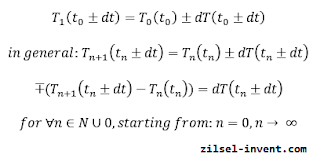## Posts

Showing posts from August, 2015

### Feel the force around you – Frictional force Part IThis is the first article in a series intended to explain the force of friction. If somebody asks you: what is the force of friction? Just give a simple answer, it is a force! Maybe it sounds crazy, but it is a 100% true answer. Let’s start thinking in equations. If we do have force on the left side of an equation that also mean that we do have a force on the right side of the equation. It is not possible to have force on the left and frogs on the right, because force and frogs are not the same things by nature. Basically, what is a force of friction? is a stupid question. The better question is: which force is the force of friction?
That is a very good question. We already started a discussion about the normal force included in the frictional force calculations. Anyway, even if a normal force is used to calculate the maximum value of the frictional force, there are a lot of differences between normal and frictional force, and also similarities. The frictional force is a reaction f…